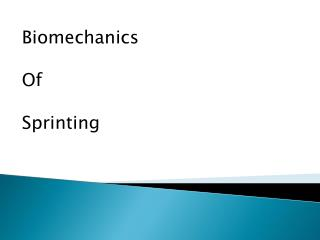DownloadDownload PresentationBiomechanics Of Sprinting

# Biomechanics Of Sprinting

Download Presentation## Biomechanics Of Sprinting

- - - - - - - - - - - - - - - - - - - - - - - - - - - E N D - - - - - - - - - - - - - - - - - - - - - - - - - - -
##### Presentation Transcript

1. Biomechanics Of Sprinting

2. Understanding Sprint Performance • Horizontal Velocity throughout the race is constantly changing. • Most Important part of the race • Acceleration Maximum velocity

3. Start • Horizontal velocity of an elite sprinter • Over 4 meters/sec at ground contact coming out of blocks • Increases to over 7 meters per second by end of the 2nd touch down • That is over half of the sprinters maximum velocity with in the first 3 steps of the start

4. Mechanics Transition • From powerful horizontal force to a more vertically directed force. • In part due to raising COG

5. Basic Mechanics • For the Start the goal is maximum horizontal force and minimizing force in all other directions • Transition phase into maximum velocity • Once maximum velocity is reached the goal is to maintain maximum velocity by producing maximum amounts of vertical force

6. Basic Mechanics • This means that Horizontal velocity is not the critical mechanical factor in sprint performance

7. Newton’s Laws • We can understand this change in focus by looking at Newton’s laws • Force= Mass(Change in velocity)/Ground time • @Start • Horizontal force=77.5*(7.0)/.60 = 905 N or 205lbs of horizontal force *77.5kg=170.8lbs

8. Newton’s Laws • @ Maximum velocity • Horizontal force= 0 @ Start Vertical Force= 77.5*(1.0)/.150= 485N or 110lbs Total Vertical Force= 750N+485N= 1235N or 277lbs

9. Newton’s Laws • As you transition into maximum velocity the horizontal force output decreases • This is not true of vertical force • Because of gravity, sprinting is a series of alternating ground and air phases

10. Newton’s Laws • To account for this the change the vertical velocity in the upward direction must increase to about .5 m/s • This is also true in the downward direction so the total vertical velocity increase equals to 1.0 m/s

11. Newton’s Laws • Vertical force= 77.5*(1.0)/.087= 890N or 199 lbs • Total Vertical Force= 759N+890N= 1,640N or 367lbs

12. Mechanics • As horizontal velocity increases the segments increase as well. • When segments increase it has a negative impact on the runners ability to produce vertical force • Another limiting factor is the body position

13. Mechanics • The body positioning of the sprinter at touchdown is actually producing horizontal braking forces • The touchdown point is actually located slightly in front of the COG • The best sprinters minimize this effect

14. Start Mechanics • Specific Performance Descriptors • Block Distances • COG Distance at Set Position • Segment Angles at Set Position • Segment Angles During Block Clearance • COG Distance at Step 1 Touchdown • Segment Angles during Step 1 • COG Distance at Step 2 touchdown • Segment Angles during Step 2

15. Critical Performance Descriptors at Maximum Velocity • Horizontal Velocity Stride Rate Stride Length Ground Contact Time Air Time Time To Maximum Upper Leg Flexion

16. Front Side/ Back Side Mechanics • The most successful sprinters focus on front side mechanics • Active recovery of the back side mechanics is important • Do not just “spin the wheels”

17. References Hunter,J., Marshall,R., McNair,P.(2005). Relationships Between Ground Reaction Force Impulse and Kinematics of Sprint-Running Acceleration. Retrieved from: Journal of Applied Biomechanics, 21,31-43 Kovacs,M. Speed Training: Linear Acceleration. Retrived from NSCA Cavagna, G., Komarek, L., Mazzoleni, S. (1971, May) The Mechanics of Sprint Running. Retrieved from: The Journal of Physiology, 217, 709-721 Mann, R. (2011). The Mechanics of Sprinting an Hurdling.